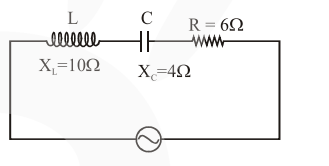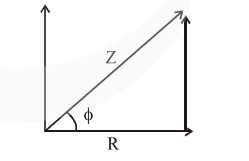# In a series LCR circuit, the inductive reactance`
Question:

In a series LCR circuit, the inductive reactance $\left(\mathrm{X}_{\mathrm{L}}\right)$ is $10 \Omega$ and the capacitive reactance $\left(\mathrm{X}_{\mathrm{C}}\right)$ is $4 \Omega$. The resistance (R) in the circuit is $6 \Omega$. The power factor of the circuit is :

1. $\frac{1}{2}$

2. $\frac{1}{2 \sqrt{2}}$

3. $\frac{1}{\sqrt{2}}$

4. $\frac{\sqrt{3}}{2}$

Correct Option: , 3

Solution:We know that power factor is $\cos \phi$,

$\cos \phi=\frac{R}{Z}$ ..........(1)

$Z=\sqrt{R^{2}+\left(X_{L}-X_{C}\right)^{2}}$ .......(2)

$(\omega \mathrm{L}-1 / \omega \mathrm{C})$$\Rightarrow Z=\sqrt{6^{2}+(10-4)^{2}}$

$\Rightarrow Z=6 \sqrt{2} \mid \cos \phi=\frac{6}{6 \sqrt{2}}$

$\cos \phi=\frac{1}{\sqrt{2}}$